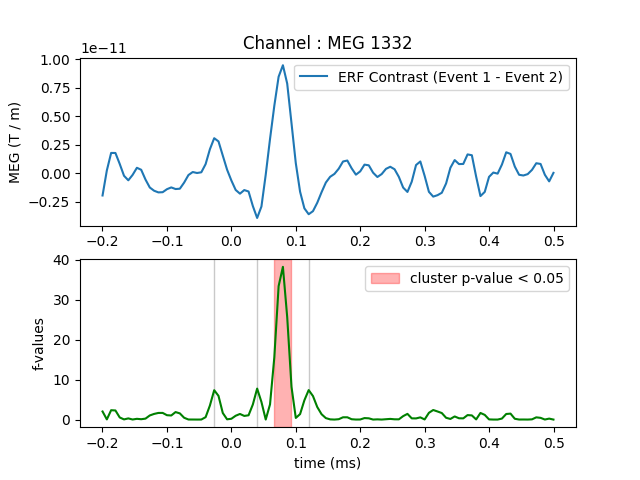# Permutation F-test on sensor data with 1D cluster level¶

One tests if the evoked response is significantly different between conditions. Multiple comparison problem is addressed with cluster level permutation test.

# Authors: Alexandre Gramfort <alexandre.gramfort@inria.fr>
#

import matplotlib.pyplot as plt

import mne
from mne import io
from mne.stats import permutation_cluster_test
from mne.datasets import sample

print(__doc__)


Set parameters

data_path = sample.data_path()
raw_fname = data_path + '/MEG/sample/sample_audvis_filt-0-40_raw.fif'
event_fname = data_path + '/MEG/sample/sample_audvis_filt-0-40_raw-eve.fif'
tmin = -0.2
tmax = 0.5

#   Setup for reading the raw data

channel = 'MEG 1332'  # include only this channel in analysis
include = [channel]


Out:

Opening raw data file /home/circleci/mne_data/MNE-sample-data/MEG/sample/sample_audvis_filt-0-40_raw.fif...
Read a total of 4 projection items:
PCA-v1 (1 x 102)  idle
PCA-v2 (1 x 102)  idle
PCA-v3 (1 x 102)  idle
Average EEG reference (1 x 60)  idle
Range : 6450 ... 48149 =     42.956 ...   320.665 secs


Read epochs for the channel of interest

picks = mne.pick_types(raw.info, meg=False, eog=True, include=include,
event_id = 1
epochs1 = mne.Epochs(raw, events, event_id, tmin, tmax, picks=picks,
baseline=(None, 0), reject=reject)
condition1 = epochs1.get_data()  # as 3D matrix

event_id = 2
epochs2 = mne.Epochs(raw, events, event_id, tmin, tmax, picks=picks,
baseline=(None, 0), reject=reject)
condition2 = epochs2.get_data()  # as 3D matrix

condition1 = condition1[:, 0, :]  # take only one channel to get a 2D array
condition2 = condition2[:, 0, :]  # take only one channel to get a 2D array


Out:

Not setting metadata
72 matching events found
Applying baseline correction (mode: mean)
4 projection items activated
Rejecting  epoch based on EOG : ['EOG 061']
Rejecting  epoch based on EOG : ['EOG 061']
Rejecting  epoch based on EOG : ['EOG 061']
Rejecting  epoch based on EOG : ['EOG 061']
Rejecting  epoch based on EOG : ['EOG 061']
Rejecting  epoch based on EOG : ['EOG 061']
Rejecting  epoch based on EOG : ['EOG 061']
Rejecting  epoch based on EOG : ['EOG 061']
Rejecting  epoch based on EOG : ['EOG 061']
Rejecting  epoch based on EOG : ['EOG 061']
Rejecting  epoch based on EOG : ['EOG 061']
Rejecting  epoch based on EOG : ['EOG 061']
Rejecting  epoch based on EOG : ['EOG 061']
Rejecting  epoch based on EOG : ['EOG 061']
Rejecting  epoch based on EOG : ['EOG 061']
Rejecting  epoch based on EOG : ['EOG 061']
73 matching events found
Applying baseline correction (mode: mean)
4 projection items activated
Rejecting  epoch based on EOG : ['EOG 061']
Rejecting  epoch based on EOG : ['EOG 061']
Rejecting  epoch based on EOG : ['EOG 061']
Rejecting  epoch based on EOG : ['EOG 061']
Rejecting  epoch based on EOG : ['EOG 061']
Rejecting  epoch based on EOG : ['EOG 061']
Rejecting  epoch based on EOG : ['EOG 061']
Rejecting  epoch based on EOG : ['EOG 061']
Rejecting  epoch based on EOG : ['EOG 061']
Rejecting  epoch based on EOG : ['EOG 061']
Rejecting  epoch based on EOG : ['EOG 061']


Compute statistic

threshold = 6.0
T_obs, clusters, cluster_p_values, H0 = \
permutation_cluster_test([condition1, condition2], n_permutations=1000,
threshold=threshold, tail=1, n_jobs=1,


Out:

stat_fun(H1): min=0.000227 max=38.167093
Running initial clustering
Found 4 clusters
Permuting 999 times...

0%|          |  : 0/999 [00:00<?,       ?it/s]
16%|#5        |  : 157/999 [00:00<00:00, 4576.20it/s]
33%|###2      |  : 325/999 [00:00<00:00, 4592.60it/s]
49%|####8     |  : 488/999 [00:00<00:00, 4603.97it/s]
65%|######5   |  : 651/999 [00:00<00:00, 4614.96it/s]
82%|########1 |  : 817/999 [00:00<00:00, 4628.78it/s]
98%|#########7|  : 979/999 [00:00<00:00, 4636.06it/s]
100%|##########|  : 999/999 [00:00<00:00, 4813.71it/s]
Computing cluster p-values
Done.


Plot

times = epochs1.times
plt.close('all')
plt.subplot(211)
plt.title('Channel : ' + channel)
plt.plot(times, condition1.mean(axis=0) - condition2.mean(axis=0),
label="ERF Contrast (Event 1 - Event 2)")
plt.ylabel("MEG (T / m)")
plt.legend()
plt.subplot(212)
for i_c, c in enumerate(clusters):
c = c
if cluster_p_values[i_c] <= 0.05:
h = plt.axvspan(times[c.start], times[c.stop - 1],
color='r', alpha=0.3)
else:
plt.axvspan(times[c.start], times[c.stop - 1], color=(0.3, 0.3, 0.3),
alpha=0.3)
hf = plt.plot(times, T_obs, 'g')
plt.legend((h, ), ('cluster p-value < 0.05', ))
plt.xlabel("time (ms)")
plt.ylabel("f-values")
plt.show()Total running time of the script: ( 0 minutes 3.590 seconds)

Estimated memory usage: 8 MB

Gallery generated by Sphinx-Gallery